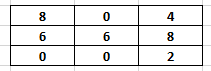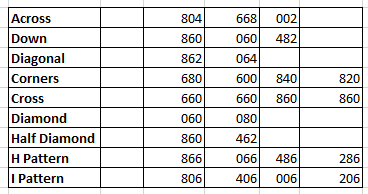# 1-2-3 Rundown• Use Lottery Math for addition and subtraction in the rundowns.
• Steps for 1-2-3 Rundown:
1. Take the recent Draw number.
2. Perform the following steps to get the first set of numbers.
• Add +1 to the first digit of the draw number
• Add +2 to the second digit of the draw number
• Add +3 to the third digit of the draw number
3. Perform the following steps to get the second set of numbers.
• Subtract 1 from the first digit of the draw number
• Subtract 2 from the second digit of the draw number
• Subtract 3 from the third digit of the draw number
4. Perform the following steps to get the third set of numbers.
• Add +3 to the first digit of the draw number
• Add +2 to the second digit of the draw number
• Add +1 to the third digit of the draw number
• After finding the 3 sets of numbers as explained above, place them one after another in a Tic-Tac-Toe (TTT) grid.
• Use the Tic-Tac-Toc (TTT) method of picking different number combinations to play for next couple of draws.

Disclaimer: Please backtest the rundown in your state to ensure it works. All rundown systems might not be working in every state.

### Example:

Step 1:  Latest Draw Number:   781

Step 2:  (Using Lottery Math)       804 (See below for the details)

• First Digit                   7 + 1 = 8
• Second Digit             8 + 2  = 0
• Third Digit                  1 + 3 = 4

Step 3: (Using Lottery Math)       668 (See below for the details)

• First Digit                   7 – 1 = 6
• Second Digit              8 – 2 = 6
• Third Digit                  1 – 3 = 8

Step 4: (Using Lottery Math)     002 (See below for the details)

• First Digit                   7 + 3 = 0
• Second Digit             8 + 2 = 0
• Third Digit                  1 + 1 = 2

Step 5: Create the TTT grid using the numbers obtained from Steps 2, 3 & 4Step 6:  Number Combinations to play from the TTT boardNote:  Please refer the TIC-TAC-TOE grid topic to understand how the numbers are derived for different patterns in the above example.